# Free GED Math Practice Test (Over 1000 Math Questions To Solve)

The GED Math practice tests has been created to help you pass the Mathematical Reasoning section of the GED.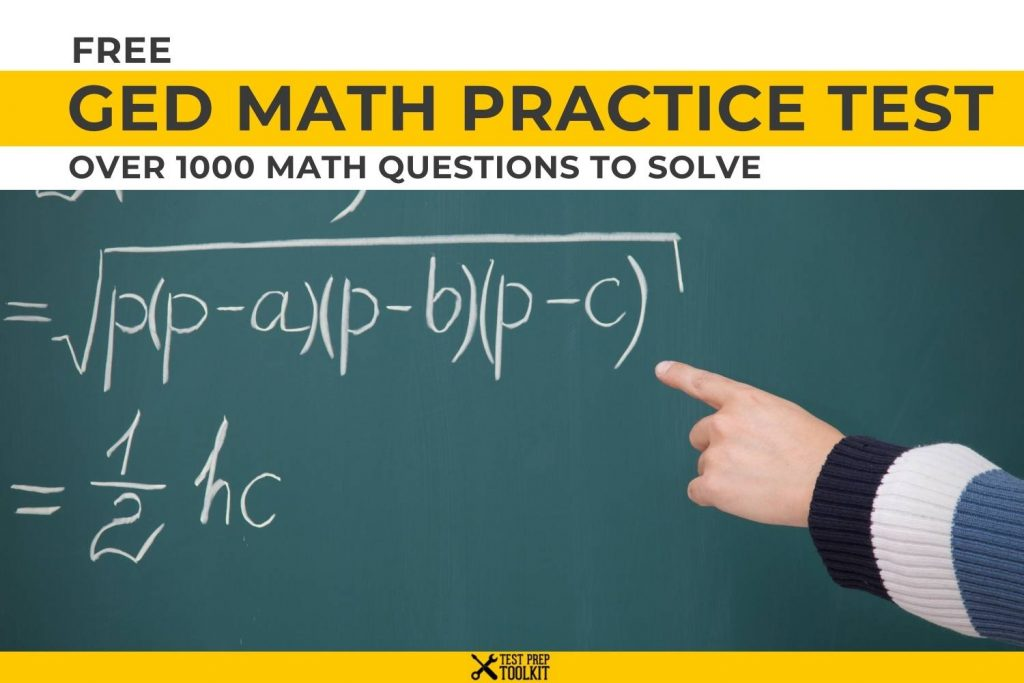Our tests to guide you comprehend the original GED Math exam you face during the GED test. You will receive detailed scoring results at the end of each exam to let you know your strengths and weaknesses.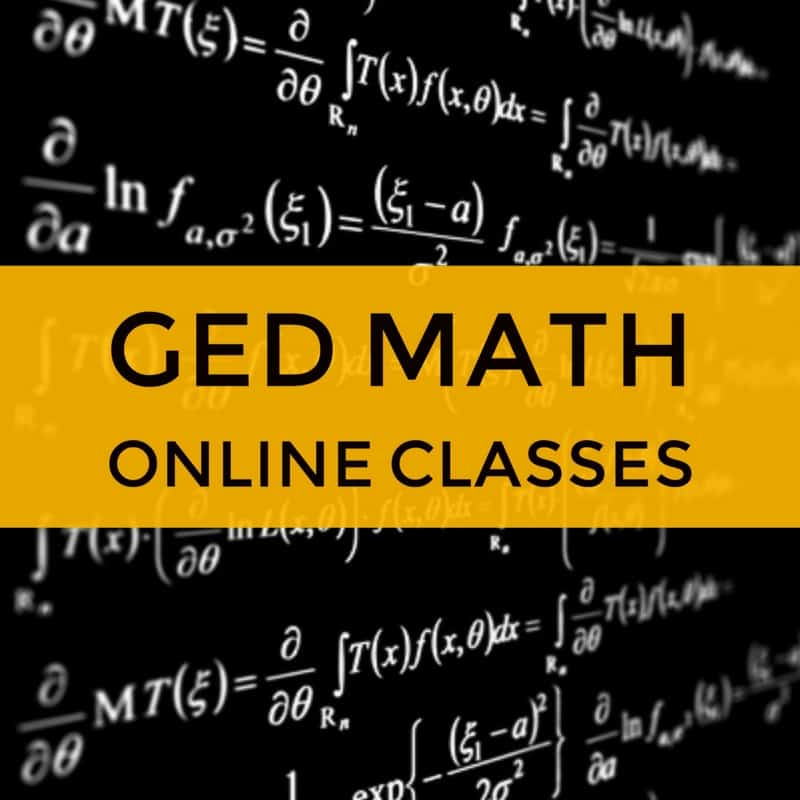GED Math Blueprint Course45 in-depth videos lessons + 3 Practice Tests (We also include 100s of Practice Questions for FREE)

This is the home of our exercise test GED. All of our exams are designed to meet the requirements of the most recent updates.

We also have GED Math Video Lessons

### A Quick Guide to GED Math Test

The GED Math test is a single section test with 46 questions. Students will be given 115 minutes to complete the test. It is divided into two parts. The beginning part, which contains the first five test questions, do not allow the use of calculators. Students will be permitted to use the calculator for part 2, which contains the remaining 41 questions.

You have the option to bring your own TI-30XS calculator to solve the question during the test or you can use the on-screen calculator. Along with the calculator, a formula sheet will also be provided on the day of the test. You may view the formula sheet here while answering our GED practice test for math. Because you can see the formulas during the test, it only means that you want to focus on understanding how to apply them instead of just merely memorizing them.

The types of items included in the Math test is multiple-choice, drag-and-drop, hot spot, and fill-in-the-blank. The questions focus on two content domains: algebraic problem-solving (55%) and quantitative problem solving (45%).

Overall, the GED Math test checks your mastery of foundation math skills. You must be able to demonstrate your skills in number sense and computation, algebraic expressions and functions, data analysis and statistics, and geometric measurement.

Full Online GED Classes Here

Quick Tips to Remember When Preparing for the GED Math Test

1. Practice!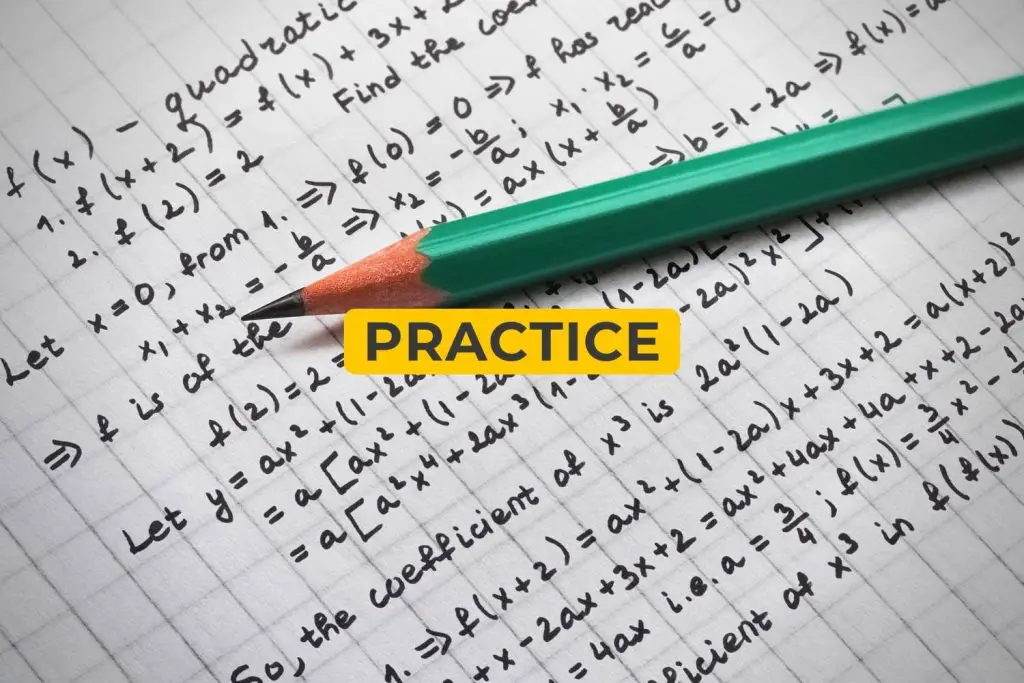Remember, the Math test only gives you 115 minutes to finish everything. So you need to work at a relatively fast pace. But you can’t perform faster if you are no longer used to doing mathematical computations. Take our GED Math practice tests now to have a high GED Math test scores. This will aid the student calculate or answer faster and more accurately.

1. Fully understand the problem.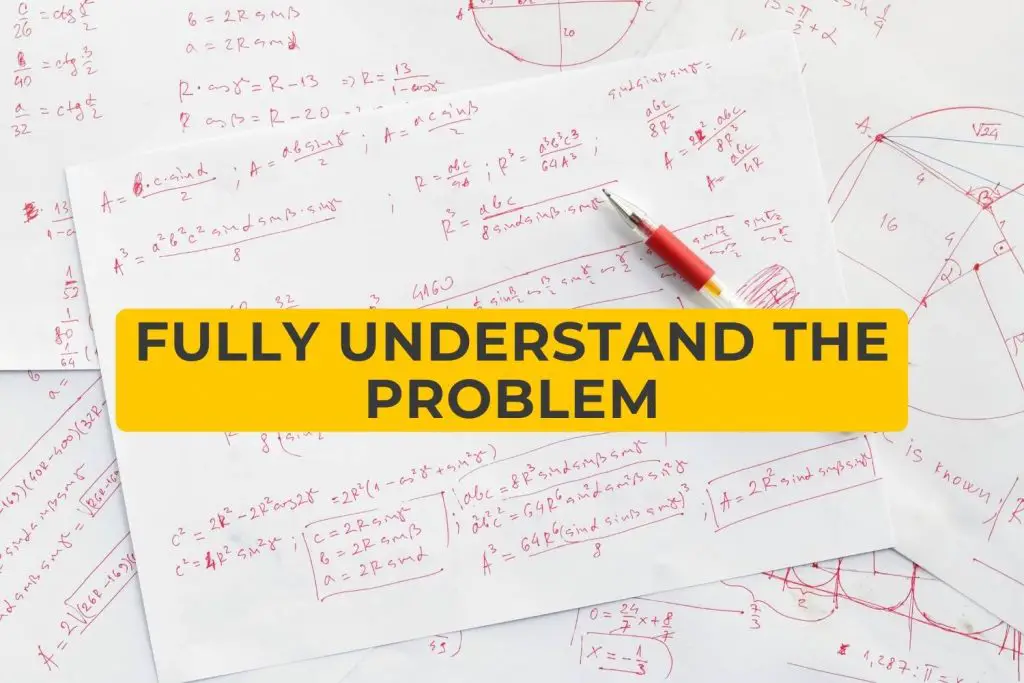Don’t compute the figures without understanding it. Focus on comprehending the question first as some items are designed to provide confusing details to test whether you really know what you’re doing.

More review materials: GED Study Guide

Check our Math Blueprint Video Course covering every possible topic for GED Math. It includes +100 videos, +2000 problems and loads of information.Compute twice before submitting your final answer. See if it is really the solution being presented to you.

1. Use all the resources you have.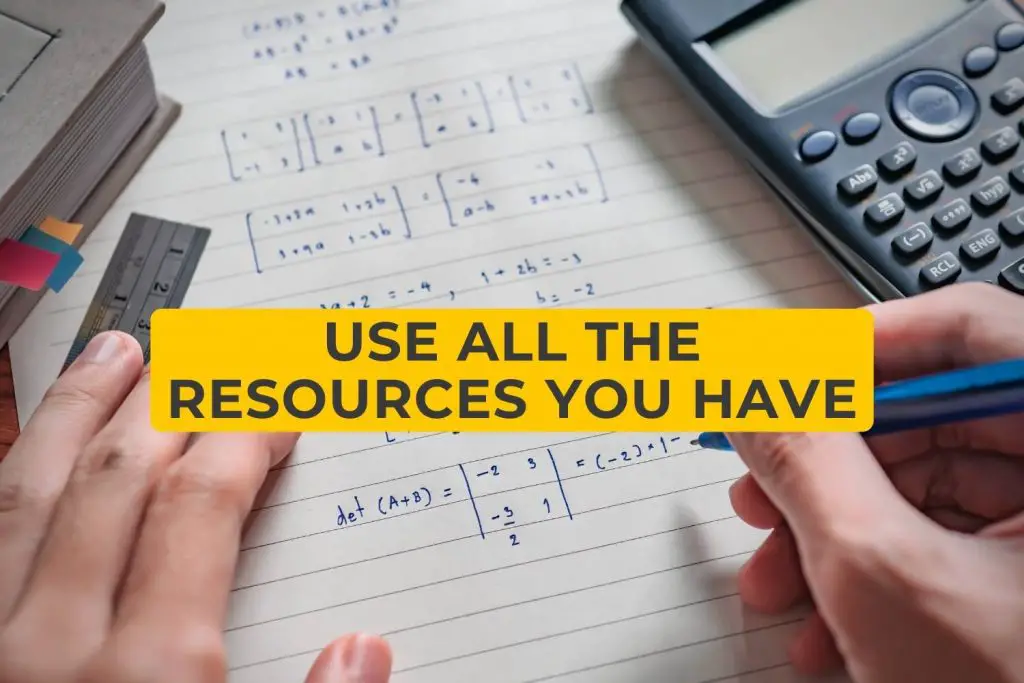You have a formula sheet and a calculator during the test. Use them to help you arrive at the correct answer. Also, it would be good to analyze the formulas and practice using them before the test. Remember, it’s not memorizing them that matters, but applying and understanding them. The best way to put these formulas to do is by taking our Math practice tests. Attending our online classes will also guide you comprehend how to use these formulas and you will have a high GED Math test score.

Take the Math practice test now. See the list of topics you need to study on our GED math page.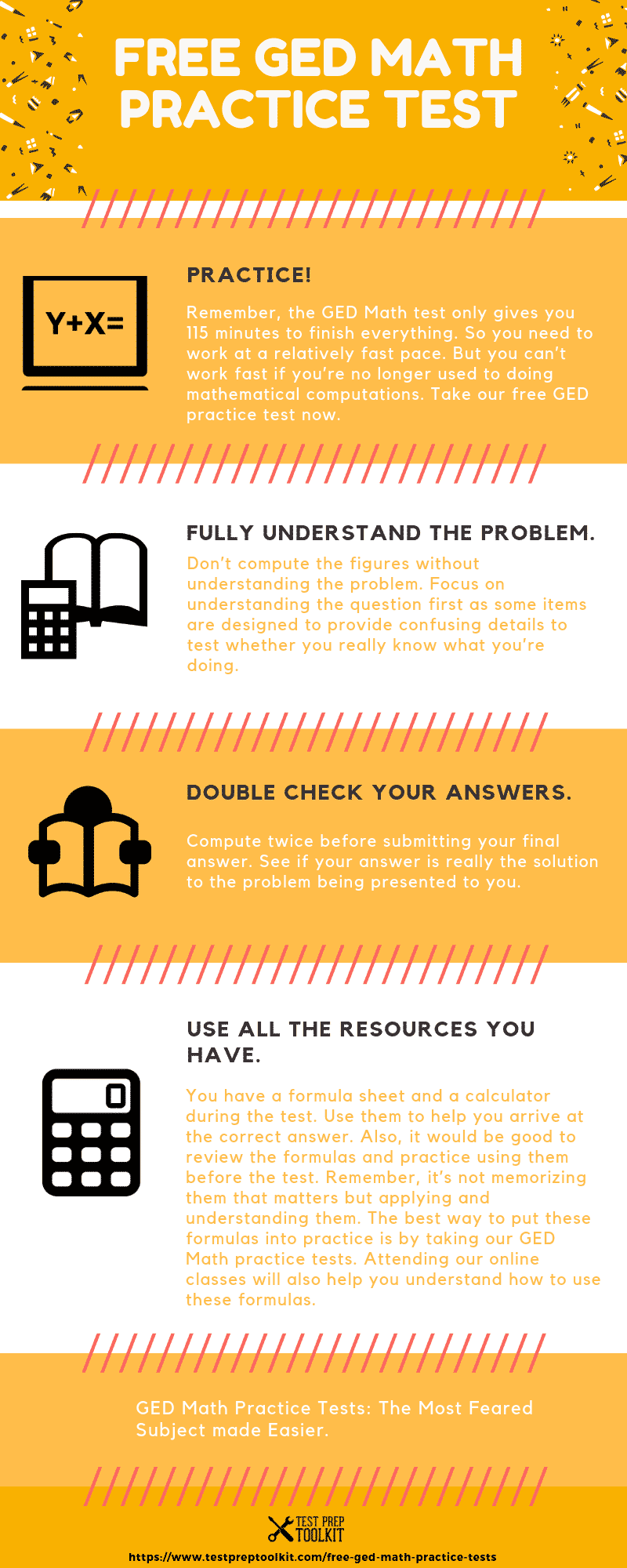Related Topics:

## Save Up To 20% Magoosh Test Prep

We’ve partnered with Magoosh Test Prep to get you EXCLUSIVE DISCOUNTS on a huge selection of test prep courses. We’ve seen a lot of students go through their programs and come out with a much higher test score than they otherwise would have.